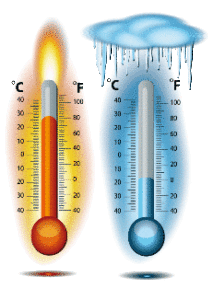# Temperature

0
1261The bodies are characterized by certain thermal states. Some bodies have lower temperatures, but some are more hot. Temperature characterizes the degree of warmth of a body. By warming up the body its temperature increases, and most often its volume. Some other characteristics of the body depends of its degree of warming.

Temperature is one of the seven basic physical quantities. In SI units, we have a thermodynamic or Kelvin scale. The unit for thermodynamic temperature is one Kelvin (1K). However in practice, the degree of Celsius is used for temperature. Kelvin’s (thermodynamic) temperature is characterized by (T), while the Celsius temperature is marked with (t). Device for temperature measurement is called thermometer. A liquid-based thermometer measures the volume so that a rise in volume indicates an increase in temperature. In practice, most commonly used thermometers filled with mercury and alcohol. The core part of the mercury thermometer is a narrow tube that has a ball or roller expansion at one end – a reservoir with mercury. The tube is closed, and there is no air above the mercury so it can spread freely. There is a temperature scale in the temperature range in the tube.Each thermometer has two characteristic points, i.e. base points.
One point corresponds to the freezing pint of the water, and another to the boiling point of the water. Both freezing and boiling water is taken under normal conditions. If the level of mercury during the freezing of water is indicated as 0 °, and the mercury level during boiling is indicated as 100 °, we get two constant temperatures. On the Celsius scale, this interval is divided into 100 equal parts and each part represents 1˚C. One Kelvin is equal to a Celsius degree. The difference is only at the beginning (zero scale). In the mid 19th century, English physicist William Thomson (Lord Kelvin) studied the spread of gases and concluded that the lowest possible temperature was -273,16˚ C, meaning that at that temperature T = 0 K. This temperature is called the absolute zero (temperature).

Temperature is one of the seven basic physical quantities.
In SI, a thermodynamic or Kelvin scale was introduced. The thermodynamic temperature unit is one Kelvin (1K). In practice, the degree of Celsius is used for temperature. Kelvin’s (thermodynamic) temperature is characterized by (T), while the Celsius temperature is marked with (t). Temperature measurement device is a thermometer. A liquid-based thermometer measures the volume so that a rise in volume indicates an increase in temperature. In practice, most commonly used thermometers filled with mercury and alcohol. The core part of the live thermometer is a narrow tube that has a ball or roller expansion at one end – a reservoir with mercury. The tube is closed, and there is no air above the air so it can spread freely. There is a temperature scale in the temperature range with the tube.

Each thermometer has two characteristic points, ie base points.
One corresponds to water haziness, and another boils water. Frost and boiling water is taken under normal conditions. If the water level is zero at 0 °, and the level of water is boiling at 100 °, we get two constant temperatures. On the Celsius scale, this interval is divided into 100 equal parts. Each part represents 1˚C.
One Kelvin is equal to a Celsius degree. The difference is only at the beginning (zero scale). In the mid-19th century, English physicist William Thomson (Lord Kelvin) studied the spread of gases and concluded that the lowest possible temperature was -273,16˚ C. Na
This degree is T = 0 K. This temperature is called the absolute zero (temperature).

[contact-form-7 id=”69″]
SHARE
Previous articleThe nature
Next articleCoulomb’s Law and Electric Field